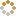﻿ N-B-U-T Online Judge :: NBUT 2015 Timed NOJ Training #003 :: Radical is lonely
• ### [B] Radical is lonely

• 时间限制: 1000 ms　内存限制: 65535 K
• 问题描述
• Radical is very lonely,he only can play with sequences just like a single dog.
He has a sequence Arr consisting of n integers. He calls a sequence Arr[i],Arr[i+1]...Arr[j](1<=i<=j<=n).The value(j-i+1)
denotes the length of the subsegment.
He want you to help him solve a problem.Your task is to find the longest subsegment of Arr, such that it is possible to change at most one number (change one number to any integer you want)
from the subsegment to make the subsegment strictly increasing.
Radical needn't the reason,he only want to get the the maximum length of the subsegment you find.
• 输入
• The first line contains integer n(1<=n<=10^5).The next line contains n integers Arr,Arr...Arr[n](1<=Arr[i]<=10^9)
• 输出
• In a single line , print the maximum length of the subsegment.
• 样例输入
• ```5
1 2 3 4 1
10
1 2 3 4 5 5 6 7 8 9```
• 样例输出
• ```5
6
```
• 提示
• `无`
• 来源
• `Radical@NBUT`
• 操作
﻿